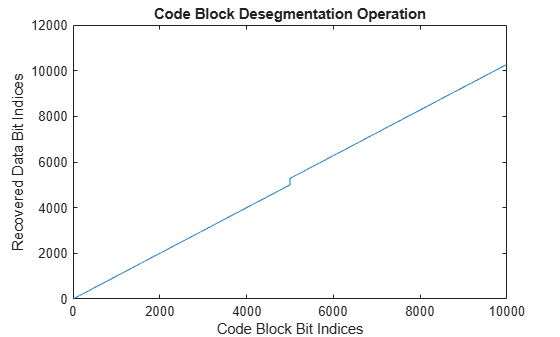nrCodeBlockDesegmentLDPC

LDPC code block desegmentation and CRC decoding

Description

example

[blk,err] = nrCodeBlockDesegmentLDPC(cbs,bgn,blklen) concatenates the input code block segments cbs into a single output data block blk of length blklen. The function validates the data dimensions of the input cbs based on the specified base graph number bgn and output block length blklen. The function removes any filler bits and type-24B cyclic redundancy check (CRC) bits present in the input cbs. The output err is the result of the type-24B CRC decoding (if applicable). This process is the inverse of the low-density parity-check (LDPC) code block segmentation specified in TS 38.212 Section 5.2.2  and implemented in nrCodeBlockSegmentLDPC.

Examples

collapse all

Perform code block segmentation of a random sequence of binary input data.

bgn = 1;
blklen = 10000;
cbs = nrCodeBlockSegmentLDPC(randi([0 1],blklen,1),bgn);

When the base graph number is 1, segmentation occurs whenever the input length is greater than 8448. The input data of length 10000 is split into two code block segments of length 5280. The code block segments have filler bits and CRC attached.

size(cbs)
ans = 1×2

5280           2

Concatenate the code block segments.

[blk,err] = nrCodeBlockDesegmentLDPC(cbs,bgn,blklen);

The concatenated result is of the same size as the original input with CRC and filler bits removed.

blkSize = size(blk)
blkSize = 1×2

10000           1

Verify if the CRC decoding was successful by checking the error vector.

err
err = 1x2 uint32 row vector

0   0

Create a matrix representing two code block segments. Each element contains the linear index of that element within the matrix.

cbs = reshape([1:10560]',[],2);

Concatenate the code block segments using the specified base graph number and output block length.

bgn = 1;
blklen = 10000;
blk = nrCodeBlockDesegmentLDPC(cbs,bgn,blklen);

To see how the input maps onto the output, plot code block segment indices relative to the corresponding indices in the concatenated input. In each code block segment, the last 280 bits represent CRC and filler bits. These additional bits are removed from the recovered data.

plot(blk);
xlabel('Code Block Bit Indices');
ylabel('Recovered Data Bit Indices');
title('Code Block Desegmentation Operation');Input Arguments

collapse all

Code block segments, specified as a real matrix. A matrix with only one column corresponds to one code block segment without CRC bits appended. If you specify a matrix with more than one column, each column in the matrix corresponds to a separate code block segment with type-24B CRC bits appended. In both cases, the code block segments can contain filler bits.

Data Types: double | int8

Base graph number, specified as 1 or 2.

Data Types: double

Output block length, specified as a nonnegative integer. If blklen is 0, then both blk and err are empty. The function uses blklen to validate the data dimensions of the input cbs and to calculate the number of filler bits to remove.

Data Types: double

Output Arguments

collapse all

Concatenated data block, returned as an empty vector (when blklen is 0) or a real column vector. The function removes any filler bits and type-24B CRC bits present in the input cbs. The output blk inherits its data type from the input cbs.

Data Types: double | int8

CRC error, returned as one of these values:

• Empty vector — The function returns this value when blklen is 0 or if cbs has only one column (CRC decoding does not take place).

• Vector of nonnegative integers — If cbs has more than one column, err contains the CRC error bits obtained from decoding the type-24B CRC bits in each code block segment. The length of err is equal to the number of code block segments (number of columns in the input cbs).

Data Types: uint32

 3GPP TS 38.212. “NR; Multiplexing and channel coding.” 3rd Generation Partnership Project; Technical Specification Group Radio Access Network.